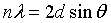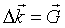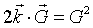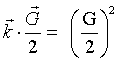PHY.K02UF Molecular and Solid State Physics

## Crystal diffraction

Everything moves like a wave and exchanges energy and momentum like a particle. When waves move through a crystal they diffract. Light, sound, neutrons, atoms, and electrons are all diffracted by crystals.

Kittel chapter 2: Crystal diffraction or R. Gross und A. Marx: Strukturanalyse mit Beugungsmethoden

For the exam
• You should know that every periodic function can be expressed as a Fourier series, f(r) = ΣfGexp(iG·r), where the G's are the reciprocal lattice vectors.
• You should be able to determine the reciprocal lattice vectors of any Bravais lattice.
• You should know that the reciprocal lattice of an orthorhombic lattice (a,b,c) is also an orhtorhombic lattice (2π/a,2π/b,2π/c); the reciprocal lattice of fcc is bcc and the reciprocal lattice of bcc is fcc.
• You should be able to construct the first Brillouin zone of any reciprocal lattice.
• You should know the different forms of the diffraction condition given in the table below.
• You should be able to explain how the Bravais lattice and the size of the unit cell can be determined in a diffraction experiment.
• You should know how to calculate structure factors. These are the complex coefficients of the Fourier series.
• You should know how the square of the structure factor can be measured in a diffraction experiment and how this information can be used to determine the basis of the crystal structure. (The basis is the pattern of atoms that are repeated at every Bravais lattice site to create the crystal.)
• You should be able to define: powder diffraction, neutron diffraction, LEED, and Ewald shphere.
 Equivalent statements of the diffraction condition Bragg's law Bragg-GleichungLaue condition Laue-BedingungDiffraction condition 1 Diffraktion Bedingung 1Diffraction condition 2 Diffraktion Bedingung 2The shape and the dimensions of the unit cell can be deduced from the position of the Bragg reflections; the content of the unit cell, on the other hand, must be determined from the intensities of the reflections.

Solid State Physics, Ibach and Lüth

• 1. A non-mathematical introduction to X-ray diffraction. C. A. Taylor
• 2. An introduction to the scope, potential and applications of X-ray analysis. M. Laing
• 3. Introduction to the Calculation of Structure Factors. S. C. Wallwork
• 4. The Reciprocal Lattice. A. Authier
• 5. Close-packed structures. P. Krishna and D. Pandey
• 6. Pourquoi les groupes de Symétrie en Cristallographie. D. Weigel
• 7. Crystal structure analysis using the `superposition' - and `complementary' - structures. L. Höhne and L. Kutchabsky
• 8. Anomalous Dispersion of X-rays in Crystallography. S. Caticha-Ellis
• 9. Rotation Matrices and Translation Vectors in Crystallography. S. Hovmöller
• 10. Metric Tensor and Symmetry Operations in Crystallography. G. Rigault
• 11. The Stereographic Projection. E. J. W. Whittaker
• 12. Projections of Cubic Crystals. Ian 0. Angell and Moreton Moore
• 13. Symmetry. L. S. Dent Glasser
• 14. Space Group Patterns. W. M. Meier
• 15. Elementary X-Ray Diffraction for Biologists. Jenny P. Glusker
• 16. The Study of Metals and Alloys by X-ray Powder Diffraction Methods. H. Lipson
• 17. An Introduction to Direct Methods. The Most Important Phase Relationships and their Application in Solving the Phase Problem. H. Schenk
• 18. An Introduction to Crystal Physics. Ervin Hartmann
• 19. Introduction to Neutron Powder Diffractometry. E. Arzi
• 20. Crystals - A Handbook for School Teachers. Elizabeth A. Wood. This classic text is now available as a Web resource in several different language translations.
• 21. Crystal Packing. Angelo Gavezzotti and Howard Flack. Includes illustrations of the two-dimensional space groups
• 22. Matrices, Mappings and Crystallographic Symmetry. Hans Wondratschek